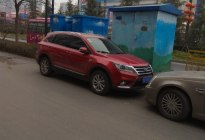### 口碑评分

[切换城市]

• 北京
• 广州
• 深圳
• 成都
• 上海
• 天津
• 重庆
• 武汉
• A
• B
• C
• F
• G
• H
• J
• L
• N
• Q
• S
• T
• X
• Y
• Z
• 直辖市：
• 北京
• 重庆
• 上海
• 天津
• A
安徽：
• 合肥
• 芜湖
• 蚌埠
• 阜阳
• 淮南
• 安庆
• 宿州
• 六安
• 淮北
• 滁州
• 马鞍山
• 铜陵
• 宣城
• 亳州
• 黄山
• 池州
• 巢湖
• B
北京：
• 北京
• C
重庆：
• 重庆
• F
福建：
• 福州
• 厦门
• 泉州
• 莆田
• 漳州
• 宁德
• 三明
• 南平
• 龙岩
• G
广东：
• 深圳
• 广州
• 东莞
• 佛山
• 中山
• 珠海
• 惠州
• 江门
• 汕头
• 湛江
• 肇庆
• 茂名
• 揭阳
• 梅州
• 清远
• 阳江
• 韶关
• 河源
• 云浮
• 汕尾
• 潮州
广西：
• 南宁
• 柳州
• 桂林
• 玉林
• 梧州
• 北海
• 贵港
• 钦州
• 百色
• 河池
• 来宾
• 贺州
• 防城港
• 崇左
贵州：
• 贵阳
• 遵义
• 黔东南
• 黔南
• 六盘水
• 毕节
• 铜仁
• 安顺
• 黔西南
甘肃：
• 兰州
• 天水
• 白银
• 庆阳
• 平凉
• 酒泉
• 张掖
• 武威
• 定西
• 金昌
• 陇南
• 临夏
• 嘉峪关
• 甘南
• H
河北：
• 石家庄
• 保定
• 唐山
• 廊坊
• 邯郸
• 秦皇岛
• 沧州
• 邢台
• 衡水
• 张家口
• 承德
• 定州
海南：
• 海口
• 三亚
• 万宁
• 文昌
• 儋州
• 琼海
• 五指山
• 东方
河南：
• 郑州
• 洛阳
• 新乡
• 南阳
• 许昌
• 平顶山
• 安阳
• 焦作
• 商丘
• 开封
• 濮阳
• 周口
• 信阳
• 驻马店
• 漯河
• 三门峡
• 鹤壁
• 济源
湖北：
• 武汉
• 宜昌
• 襄阳
• 荆州
• 十堰
• 黄石
• 孝感
• 黄冈
• 恩施
• 荆门
• 咸宁
• 鄂州
• 随州
• 潜江
• 天门
• 仙桃
• 神农架
湖南：
• 长沙
• 株洲
• 益阳
• 常德
• 衡阳
• 湘潭
• 岳阳
• 郴州
• 邵阳
• 怀化
• 永州
• 娄底
• 湘西
• 张家界
黑龙江：
• 哈尔滨
• 大庆
• 齐齐哈尔
• 牡丹江
• 绥化
• 佳木斯
• 鸡西
• 双鸭山
• 鹤岗
• 黑河
• 伊春
• 七台河
• 大兴安岭
• J
江苏：
• 苏州
• 南京
• 无锡
• 常州
• 徐州
• 南通
• 盐城
• 淮安
• 连云港
• 泰州
• 宿迁
• 镇江
江西：
• 南昌
• 赣州
• 九江
• 宜春
• 吉安
• 上饶
• 萍乡
• 抚州
• 景德镇
• 新余
• 鹰潭
吉林：
• 长春
• 吉林
• 四平
• 延边
• 松原
• 白城
• 通化
• 白山
• 辽源
• L
辽宁：
• 沈阳
• 大连
• 鞍山
• 锦州
• 抚顺
• 营口
• 盘锦
• 朝阳
• 丹东
• 辽阳
• 本溪
• 葫芦岛
• 铁岭
• 阜新
• N
内蒙古：
• 呼和浩特
• 包头
• 赤峰
• 鄂尔多斯
• 通辽
• 呼伦贝尔
• 巴彦淖尔
• 乌兰察布
• 锡林郭勒盟
• 兴安盟
• 乌海
• 阿拉善盟
宁夏：
• 银川
• 吴忠
• 石嘴山
• 中卫
• 固原
• Q
青海：
• 西宁
• 海西
• 海北
• 果洛
• 海东
• 黄南
• 玉树
• 海南
• S
山西：
• 太原
• 临汾
• 大同
• 运城
• 晋中
• 长治
• 晋城
• 阳泉
• 吕梁
• 忻州
• 朔州
上海：
• 上海
山东：
• 青岛
• 济南
• 烟台
• 潍坊
• 临沂
• 淄博
• 济宁
• 泰安
• 聊城
• 威海
• 枣庄
• 德州
• 日照
• 东营
• 菏泽
• 滨州
• 莱芜
四川：
• 成都
• 绵阳
• 德阳
• 南充
• 宜宾
• 自贡
• 乐山
• 泸州
• 达州
• 内江
• 遂宁
• 攀枝花
• 眉山
• 资阳
• 凉山
• 广元
• 雅安
• 巴中
• 阿坝
• 甘孜
陕西：
• 西安
• 咸阳
• 宝鸡
• 渭南
• 汉中
• 榆林
• 延安
• 安康
• 商洛
• 铜川
• T
天津：
• 天津
• X
西藏：
• 拉萨
• 日喀则
• 山南
• 林芝
• 昌都
• 那曲
• 阿里
新疆：
• 乌鲁木齐
• 昌吉
• 巴音郭楞
• 伊犁
• 阿克苏
• 喀什
• 哈密
• 克拉玛依
• 博尔塔拉
• 吐鲁番
• 和田
• 石河子
• 克孜勒苏
• 阿拉尔
• 五家渠
• 图木舒克
• Y
云南：
• 昆明
• 曲靖
• 大理
• 红河
• 玉溪
• 丽江
• 文山
• 楚雄
• 西双版纳
• 昭通
• 德宏
• 普洱
• 保山
• 临沧
• 迪庆
• 怒江
• Z
浙江：
• 杭州
• 宁波
• 温州
• 金华
• 嘉兴
• 台州
• 绍兴
• 湖州
• 丽水
• 衢州
• 舟山
• 义乌

7.98-11.68万

• 全部在售
• 停售
筛选条件： 2017款手动变速箱(MT)1.5T重置筛选
2017款车型综合评分车主购车价车主平均油耗
2017款 1.5T 手动智能型 (查看1条口碑)8.66万起 /100km
×

### 幻速S6车主口碑

口碑类别
不限
最满意
最不满意
空间
动力
操控
油耗
舒适性
外观
内饰
性价比机智的百合
2019-02-26发表

### 1.5T 手动智能型

综合评分：5.0分
空间
5.0分
动力
5.0分
操控
5.0分
油耗
5.0分
舒适性
5.0分
外观
5.0分
性价比
5.0分最满意最满意的就是外观时尚耐看，和丰富的配置，以及内部空间了，说实话内部空间真的非常大，特别是后排纯平的地板，让后排乘坐起来更舒服！动力完全够用，加速超车不费劲!
最不满意要说有什么不满意的，那就是车子的密封不好，打开车门里面边缘总是有灰尘，后期得自己加装密封条才行
乘坐空间很大非常满意，特别是后排的纯平的地板，为后排乘客提供了非常好乘坐感受
起步只要稍微给点油，还是非常轻松的，高速超车没有任何压力
转向精准，指向明确，电子助力很轻盈，随着车速的提高，方向盘的力度也会随着变重，感觉抓地力很好，跑个一百六七，根本感觉不到车子飘!底盘偏向于舒适，非常不错，高速入弯，底盘的支撑，还是非常不错的
油耗真的不高，百公里8L，比起哈弗，实在太省油了
座椅软硬刚刚好，腰部支撑包裹也非常不错，乘坐空间真的非常舒服，减震调教的偏向于舒适，对于道路上的细小颠簸处理的很好，完全不会传递到车内，过减速带感觉底盘还是非常扎实整洁的，完全没有松动的感觉
外观时尚霸气耐看，怎么看都是那么漂亮那么霸气十足，车身侧面的腰线与门把手完美融合，修长的车身加上溜背造型，整车看上去动力十足，充满了运动气息，圆润饱满的大臀部，看上去那么漂亮性感
说实话，在这个级别里，s6的内饰用料是非常厚道的，手能摸到的地方都是软质包裹，中控台上的按键，使用起来手感也非常喜欢
性价比不用说，在这个级别里也算是数一数二的了，十万块钱，就能买的25万甚至于30的车子才能有的配置，这性价比还不高吗？买这车还不划算吗？
*上述内容的版权归发帖人和买车网所有，未经允许不得使用、转载